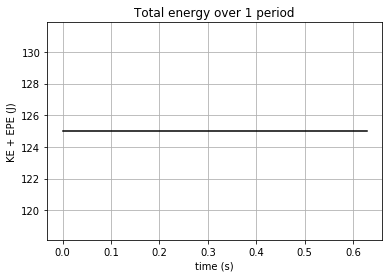# Work and Energy#

import numpy as np
%matplotlib inline
import matplotlib.pyplot as plt
from matplotlib import animation, rc
from IPython.display import HTML


## Work-energy theorem#

Mathematically, work-energy theorem is stated as the following:

$W_{12}=\int_{x_1}^{x_2}F(x)\,dx$

where $$W_{12}$$ is the work done on a body by the force $$F$$ as the body moves from point $$x_1$$ to $$x_2$$.

$$W_{12}$$ is equal to the change in energy when the body moves from $$x_1$$ to $$x_2$$.

## Forms of energy#

### Kinetic energy#

$KE=\frac{1}{2}mv^2$

where $$m$$ is mass, $$v$$ is velocity

### Potential energy#

#### Gravitational potential energy#

$GPE(x)=mgx$

where $$m$$ is mass, $$g$$ is gravitational acceleration, $$x$$ is the height above a reference point where $$x=0$$

#### Elastic potential energy#

$EPE(x)=\frac{1}{2}kx^2$

Note: total energy is constant over time

### Equipartition of Energy#

The principle of equipartition of energy states that at thermal equilibrium, magnitude of different forms of energy are equal on average.

## Tutorial Problem 3.6#

Consider a body connected to a spring, oscillating according to $$x(t)=x_0\cos(\sqrt{\frac{k}{m}}t)$$.

Derive expressions for the elastic potential energy of the body as a function of time, for the kinetic energy of the body as a function of time, and for the total energy of the body as a function of time.

Draw a simple sketch of these energies as a function of time. What is the average value of $$KE$$, and the average value of $$EPE$$, over one period? You should find that the average values of $$KE$$ and $$EPE$$ are equal.

g = 9.81 # m/s2

# input arbitrary numbers
m = 10 # mass kg
k = 1e3 # spring constant N/m
x0 = 0.5 # m

T = 2 * np.pi * np.sqrt(m/k) # period in simple harmonic motion

print("Period = %.2f" % T)

t = np.linspace(0, T, 500)

X = x0 * np.cos(np.sqrt(k/m)*t)

dxdt = -x0 * np.sqrt(k/m) * np.sin(np.sqrt(k/m)*t)

epe = 0.5 * k * X**2

ke = 0.5 * m * dxdt**2

average_epe = np.sum(epe)/len(epe)

average_ke = np.sum(ke)/len(ke)

print("Average EPE = %.dJ" % (average_epe))
print("Average KE = %.dJ" % (average_ke))

# plot figures of EPE, KE, and total energy over 1 period

fig = plt.figure(figsize=(6,4))

plt.plot(t, ke+epe, 'k')
plt.xlabel('time (s)')
plt.ylabel('KE + EPE (J)')
plt.title('Total energy over 1 period')
plt.grid(True)

plt.show()

Period = 0.63
Average EPE = 62J
Average KE = 62Jnframes = len(t)

# Plot background axes
fig, axes = plt.subplots(1,3, figsize=(15, 10))

line1, = axes.plot([], [], 'ko', lw=2)
line2, = axes.plot([], [], 'ro', lw=2)
line3, = axes.plot([], [], 'bo', lw=2)

axes.set_xlim(-0.1, 0.1)
axes.set_ylim(-0.6, 0.6)
axes.set_ylabel('displacement (m)')
axes.set_title('Motion of body over 1 period')
axes.set_xlim(-0.1, 0.1)
axes.set_ylim(0, 130)
axes.set_ylabel('EPE (J)')
axes.set_title('Elastic potential energy over 1 period')
axes.set_xlim(-0.1, 0.1)
axes.set_ylim(0, 130)
axes.set_ylabel('KE (J)')
axes.set_title('Kinetic energy over 1 period')

lines = [line1, line2, line3]

# Plot background for each frame
def init():
for line in lines:
line.set_data([], [])
return lines

# Set what data to plot in each frame
def animate(i):

x1 = 0
y1 = X[i]
lines.set_data(x1, y1)

x2 = 0
y2 = epe[i]
lines.set_data(x2, y2)

x3 = 0
y3 = ke[i]
lines.set_data(x3, y3)

return lines

# Call the animator
anim = animation.FuncAnimation(fig, animate, init_func=init,
frames=nframes, interval=10, blit=True)

HTML(anim.to_html5_video())